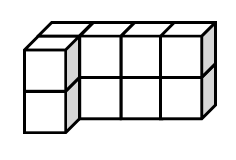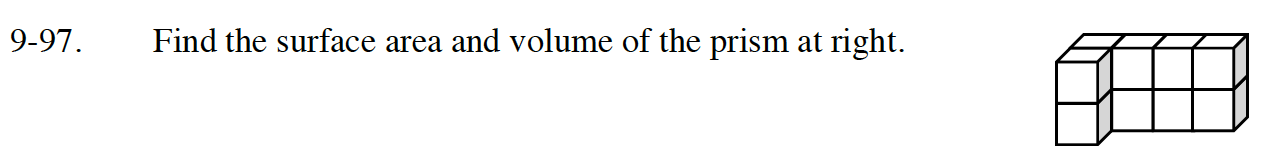Home > CC3 > Chapter 9 > Lesson 9.2.3 > Problem9-97

9-97.Find the surface area and volume of the prism at the right. Homework Help ✎Surface area is the total area of the surfaces of an object.

Volume is the space that an object occupies:
To find the volume of a prism, multiply the volume of one layer by the number of layers.

10 cubes make up this object.
How many sides act as a surface for the object and count towards the surface area?

Surface Area = 34 square units
Volume = 10 cubic units